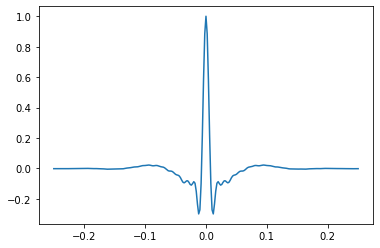# bruges is a#In other words, it’s just a load of functions that implement important equations in (mostly seismic) geophysics, from Aki-Richards to Zoeppritz.

## Quick start#

To install `bruges`, simply type the following into a terminal:

```pip install bruges
```

To create an Ormsby wavelet:

```from bruges.filters import ormbsy
w, t = ormsby(duration=0.5, dt=0.002, f=[5, 10, 40, 80])
```

The function passes back the wavelet amplitude samples `w` and the time of each sample, `t`. You can plot the wavelet with `matplotlib` like so:

```import matplotlib.pyplot as plt
plt.plot(t, w)
```Other resources